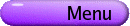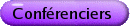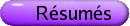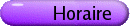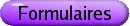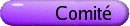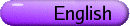Topologie générale et ses applications
Org: A. Karassev (Nipissing), M. Tuncali (Nipissing), E.D. Tymchatyn (Saskatoon) et V. Valov (Nipissing)
[PDF]

TARAS BANAKH, Nipissing University, North Bay, Canada and Lviv National University, Lviv, Ukraine
Selection Principles and Games on Multicovered Spaces
[PDF]

We shall discuss some Selection Principles and related games on multicovered spaces. A multicovered space is a pair (X,m) consisting of a set X and a family m of covers of X. The category of multicovered space is a natural place where Theory of Selection Principles develops naturally and deeply. A typical selection principle asserts that for each sequence (un)n Î w Î mw of covers of a multicovered space (X,m) it is possible to select a cover v = {Bn : n Î w} of X by un-bounded subsets Bn Ì X so that for each point x Î X the index set {n Î w: x Î Bn} is "large" in a suitable sense. If "large" means "non-empty" (resp. "coinfinite") then we obtain the classical Menger (resp. Hurewicz) property. The (non-trivial and highly fruitful) interplay between Selection Principles and recently created Theory of Semifilters will be discussed as well.

NIKOLAY BRODSKIY, University of Tennessee, Knoxville, TN
Compression of uniform embeddings into Hilbert space
[PDF]

The notion of uniform embedding of metric spaces plays an important role in study of large scale properties of finitely generated groups. A map f: X® Y of metric spaces (X,dX) and (Y,dY) is called a uniform embedding if there are two real functions r- and r+ with limr®¥ r- (r) = +¥ such that r- (dX(x,z)) £ dY (f(x),f(z)) £ r+(dX(x,z)) for all x,z Î X. For example, a bi-Lipschitz map is a uniform embedding with linear functions r- and r+. If one tries to embed a given space X uniformly into Hilbert space, how close to bi-Lipschitz could the embedding be? We answer this question for finite dimensional CAT(0) cube complexes and for hyperbolic groups with word metric.

MAXIM BURKE, University of Prince Edward Island, Charlottetown, PE C1A 4P3
Large entire cross-sections of second category sets in Rn+1
[PDF]

By the Kuratowski-Ulam theorem, if A Í Rn+1 = Rn ×R is a Borel set which has second category intersection with every ball (i.e., is "everywhere second category"), then there is a y Î R such that the section AÇ(Rn ×{y}) is everywhere second category in Rn ×{y}. If A is not Borel, then there may not exist a large cross-section through A, even if the section does not have to be flat. For example, a variation on a result of T. Bartoszynski and L. Halbeisen shows that there is an everywhere second category set A Í Rn+1 such that for any polynomial p in n variables, AÇgraph(p) is finite. It is a classical result that under the Continuum Hypothesis, there is an everywhere second category set L in Rn+1 which has only countably many points in any first category set. In particular, LÇgraph(f) is countable for any continuous function f :Rn ® R. We prove that it is relatively consistent with ZFC that for any everywhere second category set A in Rn+1, there is a function f : Rn ®R which is the restriction to Rn of an entire function on Cn and is such that, relative to graph(f), the set AÇgraph(f) is everywhere second category. Moreover, given a non-negative integer k, a function g : Rn® R of class Ck and a positive continuous function e: Rn ® R, we may choose f so that for all multiindices a of order at most k and for all x Î Rn, |Da f(x)-Da g(x)| < e(x). The method builds on fundamental work of K. Ciesielski and S. Shelah which provides, for everywhere second category sets in 2w×2w, large sections which are the graphs of homeomorphism of 2w.

ROBERT CAUTY, Université Paris 6, 4 place Jussieu, 75252 Paris Cedex 05
Approximation by chain mappings and fixed points
[PDF]

We use approximations of multivalued maps by chain mappings into singular chain complexes to prove fixed point theorems.

DOUGLAS CHILDERS, University of Alabama at Birmingham
Sequences of rotation numbers determine degeneracy of a lamination on the closed unit disk
[PDF]

Let S1 = R/Z denote the complex unit circle and define s: S1 ® S1 by s(t) = 2t mod 1. Thurston describes a collection of s-invariant laminations on the complex unit disk [`(D)], which gives a combinatoric parametrization of the Mandelbrot set. Each one of these laminations defines an equivalence relation ~ on S1 such that (s, ~ ) induces a map F : S1/ ~  ® S1/ ~ . Often, there exists a quadratic polynomial P with Julia set J such that P|J is semi-conjugate to F. However there are obstructions to this being true in general. One of these obstructions is that S1/ ~ could reduce to a point. In this case we call the lamination degenerate.

Bullett and Sentenac introduced the notion of a closed set having a sequence of rotation numbers for s. This notion is related to "ouady tuning". We use this concept to give a necessary and sufficient condition for when the lamination is degenerate.

DALE DANIEL, Lamar University
Applications of selections to the Hahn-Mazurkiewicz Problem
[PDF]

The Hahn-Mazurkiewicz Problem asks for conditions under which a Hausdorff space is the continuous image of a generalized arc. The first characterizations of continuous images of non-metric arcs were given by Bula and Turzanski and by Nikiel. Additional results include those of Mardesi\'c, Treybig, and many others.

In a related study, we herein consider applications of selections (carriers) to the study of images of ordered compacta. In particular, let X be a compact ordered space, Y a Hausdorff space, and let F(Y) denote the family of all nonempty closed subsets of Y with the Vietoris topology. Assuming G : X® F(Y) is continuous, we consider conditions under which G can be "lifted" to a continuous map of X onto Y.

This work relies heavily on work of R. S. Countryman as well as the theory of selections and that of continuous images of ordered compacta.

DIKRAN DIKRANJAN, Udine University
Compact groups without endomorphisms with infiniteentropy
[PDF]

Let E denote the class of compact groups that admit no continuous endomorphism with infinite topological entropy.

(1) There exist connected infinite-dimensional groups in E, but all abelian groups in E are finite-dimensional.
(2) An abelian compact connected K group belongs to E iff K is finite-dimensional.
The non-connected groups in E are hard to describe even in the abelian case. In particular, we do not know the answer to the following question: does there exist an abelian totally disconnected group in E that has endomorphisms with positive entropy?

XABIER DOMINGUEZ, Universidad de A Coruña, Campus de Elviña s/n, 15071 A Coruña, Spain
Asterisk topologies on the direct sum of topological Abelian groups
[PDF]

Let (Gi)i Î I be a family of topological Abelian groups and let Åi Î I Gi denote their algebraic direct sum, that is, the subgroup of the product Õi Î I Gi formed by those families x = (x(i))i Î I for which x(i)=0 for all but finitely many i Î I. If we let HÙ denote the character group of the Abelian group H, there are canonical algebraic isomorphisms

 æè Õ i Î I Gi öø Ù » Å i Î I GiÙ æè Å i Î I Gi öø Ù » Õ i Î I GiÙ.

Two significant group topologies arise on Åi Î I Gi in a very natural way: the box topology Tb, given by "rectangular" neighborhoods of zero, and the coproduct topology Tf, which is the finest group topology on Åi Î I Gi which makes all canonical inclusions continuous.

Nevertheless, in general none of them turns the above-mentioned isomorphisms into topological ones, when considering Tychonoff topology on the products, and compact-open topology on all dual groups. The analysis of such duality properties leads to the definition of an intermediate topology T*, the so-called asterisk topology, firstly introduced by Kaplan in 1948. Actually the lack of a natural generalization of Minkowski functional to groups gives rise to a number of variants of Kaplan's original definition. We shall survey what is known about the conditions under which such topologies are in fact the same, their behaviour with respect to duality, reflexivity and local quasi-convexity, and their relation with each other and with the box and coproduct topologies.

JERZY DYDAK, University of Tennessee
Extensions of maps to the projective plane
[PDF]

It is proved that for a 3-dimensional compact metrizable space X the infinite real projective space RP¥ is an absolute extensor of X if and only if the real projective plane RP2 is an absolute extensor of X.

VITALI FEDORCHUK, Moscow State University, Moscow, Russia
Some new applications of resolutions
[PDF]

The method of resolutions was introduced in  (see also ). This method allows us to construct new spaces using given collections of spaces. Many examples of applications of this method are given in . By applying iterated resolutions and fully closed mappings one can obtain more sophisticated examples . Here we present several new applications of resolutions.

Theorem 1 For any prime p there exists a 2-dimensional homogeneous separable first countable compact space Tp such that dim(Tp×Tq) = 3 for p ¹ q.

Question 1 Are there homogeneous metrizable compacta X and Y such that dim(X×Y) < dimX + dimY?

Recent results by J. L. Bryant  imply that if X and Y are homogeneous metrizable ANR-compacta, then

 dim(X×Y) = dimX + dimY
(1)

Question 2 Does the equality (1) hold if X is a homogeneous ANR-compactum and Y is an arbitrary (homogeneous) metrizable compactum?

Remark 1 As for Question 1, we cannot omit homogeneity of Y, since Pontryagin's surface P2 is homogeneous.

Another two results are joint with A. V. Ivanov and J. van Mill.

Theorem 2 [CH; ] For every n Î N, there exists a family of separable compacta Xi, i Î N, such that for every non-empty finite subset M of N and every non-empty closed subset F of Pi Î M Xi we have dimF = k(F)n, where k(F) is integer such that k(F) ³ 1 for infinite F. Moreover, |F|=2c for infinite closed F.

Theorem 3 [CH; ] There exists an infinite separable compactum X such that for any positive integer m, if F is an infinite closed subset of Xm, then |F|=2c and F is strongly infinite-dimensional.

Question 3 Does there exist in ZFC an n-dimensional compactum Yn, n ³ 2, such that for every m ³ 2, every non-empty closed subset F of Ymn has dimension kn, where k is some integer between 0 and m?

Question 4 Does there exist in ZFC an infinite-dimensional compactum Z such that for every non-empty closed subset F of Z2 we have either dimF = 0 or F is infinite-dimensional?

## References


V. V. Fedorchuk, Bicompacta with non-coinciding dimensionalities. Soviet Math. Dokl. 9(1968), 1148-1150.


V. V. Fedorchuk and K. P. Hart, d-23 Special Constructions. In: Encyclopedia of General Topology (K. P. Hart, J. Nagata and J. E. Vaughan, eds.), Elsevier Science Ltd., 2004, 229-232.


S. Watson, The construction of topological spaces: planks and resolutions. In: Recent Progress in General Topology (M. Husek and J. van Mill, eds.), North-Holland Publishing Co., Amsterdam, 1992, 673-757.


V. V. Fedorchuk, Fully closed mappings and their applications. (Russian) Fundament. i Prikl. Matem. (4) 9(2003), 105-235; J. Math. Sci. (New York), to appear.


J. L. Bryant, Reflections on the Bing-Borsuk conjecture. Preprint, 2003, 1-4.


V. V. Fedorchuk, A. V. Ivanov and J. van Mill, Intermediate dimensions of products. Topology Appl., submitted.

GARY GRUENHAGE, Auburn University, Auburn, AL, USA
The Baire property of function spaces with the compact-open topology
[PDF]

We discuss some recent results on the topic of the title.

VALENTIN GUTEV, University of KwaZulu-Natal
Completeness, sections and selections
[PDF]

As a rule, most of the classical Michael-type selection theorems for the existence of single-valued continuous selections are analogues and, in certain respects, generalizations of ordinary extension theorems. In contrast to this, most of the selection theorems for the existence of semi-continuous set-valued selections seem to have no proper analogues in the extension theory. In this talk, we will discuss the role of the "selection" condition in such theorem, and how it is related to the metrizability of the range of the corresponding set-valued mappings.

KAZUHIRO KAWAMURA, Institute of Mathematics, University of Tsukuba
Continuous (approximate) roots of continuous functions on compacta
[PDF]

For a compact Hausdorff space X, C(X) denotes the ring of all continuous complex-valued functions on X. The ring C(X) is said to be algebraically closed if each monic polynomial with C(X)-coefficients has a root in C(X). Starting with a classical theorem due to Countryman, Jr., we discuss a problem on topological characterizations of X with C(X) being algebraically closed. Also the existence of "approximate roots" and related topics will be discussed.

The present talk is based on joint works with A. Chigogidze, A. Karasev, T. Miura and V. Valov.

KRYSTYNA KUPERBERG, Auburn University, Auburn, AL  36849, USA
Wild and 2-wild trajectories
[PDF]

A trajectory of a flow on a 3-manifold is wild if the closure of at least one of the semi-trajectories is a wild arc. A trajectory is 2-wild if the closure of each semi-trajectory is a wild arc.

We describe a method of embedding wild trajectories in flows on 3-manifolds. This method yields interesting examples of dynamical systems. In particular, every boundary-less 3-manifold admits a flow with a discrete set of fixed points and such that the closure of every non-trivial trajectory is 2-wild, which answers a question posed at the 2004 Spring Topology and Dynamics Conference.

JOHN C. MAYER, University of Alabama at Birmingham
Thurston laminations of the unit disk, equivalence relations, and polynomial Julia sets
[PDF]

In a widely circulated preprint (1984) William Thurston introduced the notion of a (geodesic) lamination of the unit disk. Laminations are combinatorial/geometric/topological objects used to study Julia sets of polynomials in analytic complex dynamics. A lamination of the unit disk is a closed collection of chords of the disk that do not cross each other (they may touch at endpoints). Consider the power map f(z)=zd, d > 1, on the unit circle; extend f linearly to the lamination (the chords). A chord is critical if its endpoints map to one point. A lamination is invariant if the collection maps to itself forward and backward, with d-many disjoint pre-images of each chord backward, and f extends linearly to a positively-oriented confluent map of the disk to itself. The plan is that

(1) a lamination is determined by `pulling back' a set of critical chords,
(2) the lamination naturally induces an equivalence relation on the unit circle,
(3) the quotient space of the circle under this equivalence relation is a topological Julia set, and
(4) the topological Julia set is dynamically (and topologically) equivalent to an analytic Julia set for some degree d polynomial.

But there are obstructions to the fulfillment of the plan. Thurston completed most of the plan for d=2, but left some questions unanswered. Moreover, fundamental questions remain unanswered for d > 2, but recent progress has been made. In particular, one obstruction is that the lamination determined by a collection of critical chords may naturally induce a degenerate equivalence relation, collapsing the circle to a point in the quotient. In this talk, we show how the obstruction arises in degree d=2, and give some insight into degree d=3 and greater. In a subsequent talk at this meeting, D. Childers provides a complete solution to when degeneracy occurs, for degree d=2, in terms of the dynamics of the critical chord, answering an implicit question of Thurston.

This talk is mostly joint work with members of the UAB Laminations Seminar: A. Blokh, L. Oversteegen, D. Childers, G. Brouwer, C. Curry, and P. Eslami.

CHRIS MOURON, Rhodes College
Periodic points of functions on simple triod-like continua
[PDF]

A continuum X is simple triod-like if for every e > 0 there exists a continuous function ge : X® T such that T is a simple triod and for every t Î T, diam((ge(t))-1 ) < e. I will discuss the techniques used in showing when a map f : X® X has a periodic point where X is a simple triod-like continuum.

LEX OVERSTEEGEN, UAB, Birmingham, AL 35294, USA
On the structure of pseudo convex sets in the sphere
[PDF]

A connected open subset U of the sphere is called pseudo convex if for all points z in U there exist at most two closest points in the boundary of U. Answering a question by David Herron and David Minda, we show that such a set has at most two boundary components. We also provide a detailed analysis of sets with this property.

BRIAN RAINES, Baylor University, Waco, TX 76798-7328
Inverse limit spaces arising from problems in economics
[PDF]

We discuss economic models for which the space of predicted future states is an inverse limit space.

JURIS STEPRANS, York University
Products of Sequential CLP-compact spaces
[PDF]

The class of spaces which have the property that every cover by clopen sets has a finite subcover was introduced by A. Sostaks. These spaces are now known as CLP-compact spaces and it has emerged that much of the interesting behaviour of this class derives from the possibility that the product of two topological spaces contains clopen sets which do not belong to the algebra generated by the product of the algebras of clopen sets in each factor. Hence the productive nature of CLP-compactness poses certain problems not occurring in the classical case. Indeed, the problem of finding weak hypotheses under which the product of CLP-compact spaces is CLP-compact should still be considered to be open even though some progress has been recorded. It will be shown that the product of finitely many sequential, CLP-compact spaces is CLP-compact.

PAUL SZEPTYCKI, York University, Toronto, ON M3J 1P3
Transversals of almost disjoint families
[PDF]

For a family of sets A, a set X and a cardinal k (usually £ w), X is said to be a k-transversal of A if X Í ÈA and 0 ¹ |aÇX| < k for each a Î A. If k=2 we will say that X is a transversal of A. X is said to be a Bernstein set for A if Æ ¹ aÇX ¹ a for each a Î A. When an almost disjoint family admits a k-transversal or a Bernstein set was first studied in  motivated mainly by applications in topology.

We consider here a weaker property:

Definition    Given a family of sets A, A is said to admit a s-transversal if A can be written as A = È{An :n Î w} such that each An admits a transversal.

The restriction that an almost disjoint family admits a transversal is quite strong and not of much interest. However, quite a wide class of almost disjoint families admit s-transversals. We consider the question when an almost disjoint family admits a s-transversal and present some examples and applications.

## References


P. Erdös and A. Hajnal, On a property of families of sets. Acta Math. Acad. Sci. Hungar. 12(1961), 87-124.